Next: Gibb's paradox Up: Applications of statistical thermodynamics Previous: Partition functions

## Ideal monatomic gases

Let us now practice calculating thermodynamic relations using the partition function by considering an example with which we are already quite familiar: i.e., an ideal monatomic gas. Consider a gas consisting ofidentical monatomic molecules of massenclosed in a container of volume. Let us denote the position and momentum vectors of the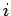th molecule byand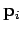, respectively. Since the gas is ideal, there are no interatomic forces, and the total energy is simply the sum of the individual kinetic energies of the molecules:(427)

where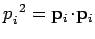.

Let us treat the problem classically. In this approach, we divide up phase-space into cells of equal volume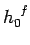. Here,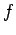is the number of degrees of freedom, andis a small constant with dimensions of angular momentum which parameterizes the precision to which the positions and momenta of molecules are determined (see Sect. 3.2). Each cell in phase-space corresponds to a different state. The partition function is the sum of the Boltzmann factor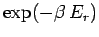over all possible states, where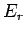is the energy of state. Classically, we can approximate the summation over cells in phase-space as an integration over all phase-space. Thus,(428)

where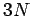is the number of degrees of freedom of a monatomic gas containingmolecules. Making use of Eq. (427), the above expression reduces to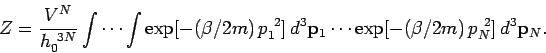(429)

Note that the integral over the coordinates of a given molecule simply yields the volume of the container,, since the energyis independent of the locations of the molecules in an ideal gas. There aresuch integrals, so we obtain the factor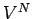in the above expression. Note, also, that each of the integrals over the molecular momenta in Eq. (429) are identical: they differ only by irrelevant dummy variables of integration. It follows that the partition function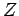of the gas is made up of the product ofidentical factors: i.e.,(430)

where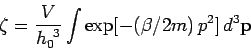(431)

is the partition function for a single molecule. Of course, this result is obvious, since we have already shown that the partition function for a system made up of a number of weakly interacting subsystems is just the product of the partition functions of the subsystems (see Sect. 7.5).

The integral in Eq. (431) is easily evaluated: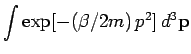(432)

where use has been made of Eq. (79). Thus,(433)

and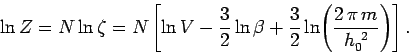(434)

The expression for the mean pressure (414) yields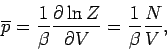(435)

which reduces to the ideal gas equation of state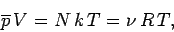(436)

where use has been made of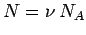and. According to Eq. (399), the mean energy of the gas is given by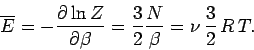(437)

Note that the internal energy is a function of temperature alone, with no dependence on volume. The molar heat capacity at constant volume of the gas is given by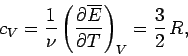(438)

so the mean energy can be written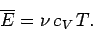(439)

We have seen all of the above results before. Let us now use the partition function to calculate a new result. The entropy of the gas can be calculated quite simply from the expression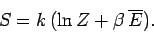(440)

Thus,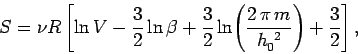(441)

or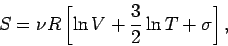(442)

where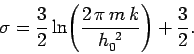(443)

The above expression for the entropy of an ideal gas is certainly new. Unfortunately, it is also quite obviously incorrect!Next: Gibb's paradox Up: Applications of statistical thermodynamics Previous: Partition functions
Richard Fitzpatrick 2006-02-02